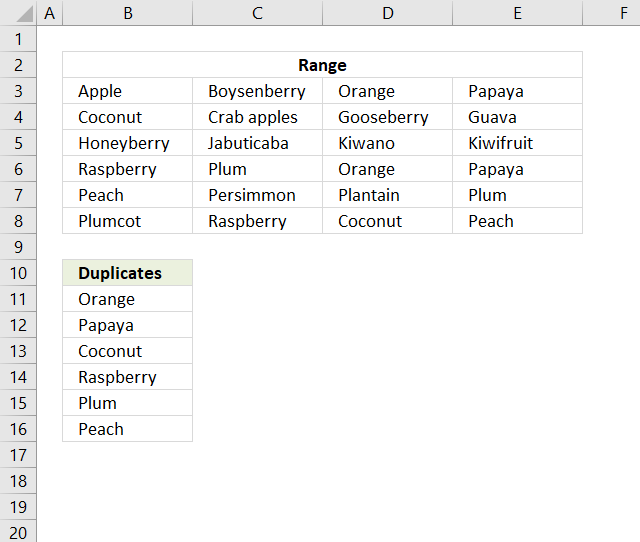Author: Oscar Cronquist Article last updated on November 21, 2018The following array formula in cell B11 extracts duplicates from cell range B3:E8, only one instance of each duplicate is returned.

Array formula in cell B11:

=INDEX(\$B\$3:\$E\$8, MIN(IF((COUNTIF(\$B\$10:B10, \$B\$3:\$E\$8)+(COUNTIF(\$B\$3:\$E\$8, \$B\$3:\$E\$8)<2))=0, ROW(\$B\$3:\$E\$8)-MIN(ROW(\$B\$3:\$E\$8))+1)), MATCH(0, (COUNTIF(\$B\$10:B10, INDEX(\$B\$3:\$E\$8, MIN(IF((COUNTIF(\$B\$10:B10, \$B\$3:\$E\$8)+IF(COUNTIF(\$B\$3:\$E\$8, \$B\$3:\$E\$8)>1, 0, 1))=0, ROW(\$B\$3:\$E\$8)-MIN(ROW(\$B\$3:\$E\$8))+1)), , 1))<>0)+(COUNTIF(\$B\$3:\$E\$8, INDEX(\$B\$3:\$E\$8, MIN(IF((COUNTIF(\$B\$10:B10, \$B\$3:\$E\$8)+IF(COUNTIF(\$B\$3:\$E\$8, \$B\$3:\$E\$8)>1, 0, 1))=0, ROW(\$B\$3:\$E\$8)-MIN(ROW(\$B\$3:\$E\$8))+1)), , 1))<2), 0))

To enter an array formula, type the formula in a cell then press and hold CTRL + SHIFT simultaneously, now press Enter once. Release all keys.

The formula bar now shows the formula with a beginning and ending curly bracket telling you that you entered the formula successfully. Don't enter the curly brackets yourself.

### Explaining formula in cell B11

This formula consists of two parts, one extracts the row number and the other the column number needed to return the correct value.

INDEX(reference, row, col)

Step 1 to 6 shows how the row number is calculated, step 7 to 11 demonstrates how to calculate the column number.

#### Step 1 - Show one instance of each duplicate

The COUNTIF function counts values based on a condition or criteria, in this case, we take into account previously displayed values in order to prevent duplicates in our output list.

COUNTIF(\$B\$10:B10, \$B\$3:\$E\$8)

becomes

COUNTIF("Duplicates", {"Apple", "Boysenberry", "Orange", "Papaya";"Coconut", "Crab apples", "Gooseberry", "Guava";"Honeyberry", "Jabuticaba", "Kiwano", "Kiwifruit";"Raspberry", "Plum", "Orange", "Papaya";"Peach", "Persimmon", "Plantain", "Plum";"Plumcot", "Raspberry", "Coconut", "Peach"})

and returns

{0,0,0,0; 0,0,0,0; 0,0,0,0; 0,0,0,0; 0,0,0,0; 0,0,0,0}

#### Step 2 - Find duplicates

(COUNTIF(\$B\$3:\$E\$8, \$B\$3:\$E\$8)<2)

becomes

({1,1,2,2;2,1,1,1;1,1,1,1;2,2,2,2;2,1,1,2;1,2,2,2}<2)

and returns

{TRUE,TRUE, FALSE,FALSE; FALSE,TRUE, TRUE,TRUE; TRUE,TRUE, TRUE,TRUE; FALSE,FALSE, FALSE,FALSE; FALSE,TRUE, TRUE,FALSE; TRUE,FALSE, FALSE,FALSE}

#### Step 3 - Add arrays

The parentheses makes sure that the arrays are added before comparing to 0 (zero).

(COUNTIF(\$B\$10:B10, \$B\$3:\$E\$8)+(COUNTIF(\$B\$3:\$E\$8, \$B\$3:\$E\$8)<2))=0

becomes

({0,0,0,0; 0,0,0,0; 0,0,0,0; 0,0,0,0; 0,0,0,0; 0,0,0,0}+{TRUE,TRUE, FALSE,FALSE; FALSE,TRUE, TRUE,TRUE; TRUE,TRUE, TRUE,TRUE; FALSE,FALSE, FALSE,FALSE; FALSE,TRUE, TRUE,FALSE; TRUE,FALSE, FALSE,FALSE})=0

becomes

{1,1,0,0;0,1,1,1;1,1,1,1;0,0,0,0;0,1,1,0;1,0,0,0}=0

and returns

{FALSE, FALSE, TRUE, TRUE;TRUE, FALSE, FALSE, FALSE;FALSE, FALSE, FALSE, FALSE;TRUE, TRUE, TRUE, TRUE;TRUE, FALSE, FALSE, TRUE;FALSE, TRUE, TRUE, TRUE}.

#### Step 4 - Replace TRUE with the corresponding row number

The IF function uses a logical expression to determine which value (argument) to return.

IF((COUNTIF(\$B\$10:B10, \$B\$3:\$E\$8)+(COUNTIF(\$B\$3:\$E\$8, \$B\$3:\$E\$8)<2))=0, ROW(\$B\$3:\$E\$8)-MIN(ROW(\$B\$3:\$E\$8))+1)

becomes

IF({FALSE, FALSE, TRUE, TRUE;TRUE, FALSE, FALSE, FALSE;FALSE, FALSE, FALSE, FALSE;TRUE, TRUE, TRUE, TRUE;TRUE, FALSE, FALSE, TRUE;FALSE, TRUE, TRUE, TRUE}, ROW(\$B\$3:\$E\$8)-MIN(ROW(\$B\$3:\$E\$8))+1)

becomes

IF({FALSE, FALSE, TRUE, TRUE;TRUE, FALSE, FALSE, FALSE;FALSE, FALSE, FALSE, FALSE;TRUE, TRUE, TRUE, TRUE;TRUE, FALSE, FALSE, TRUE;FALSE, TRUE, TRUE, TRUE}, {1;2;3;4;5;6})

and returns

{FALSE,FALSE, 1,1; 2,FALSE,FALSE,FALSE; FALSE,FALSE,FALSE,FALSE; 4,4,4,4; 5,FALSE,FALSE,5; FALSE,6,6,6}

#### Step 5 - Find smallest value

The MIN function finds the minimum value in the array ignoring the boolean values.

MIN(IF((COUNTIF(\$B\$10:B10, \$B\$3:\$E\$8)+(COUNTIF(\$B\$3:\$E\$8, \$B\$3:\$E\$8)<2))=0, ROW(\$B\$3:\$E\$8)-MIN(ROW(\$B\$3:\$E\$8))+1))

becomes

MIN({FALSE,FALSE, 1,1; 2,FALSE,FALSE,FALSE; FALSE,FALSE,FALSE,FALSE; 4,4,4,4; 5,FALSE,FALSE,5; FALSE,6,6,6})

and returns 1. This tells us that the first duplicate value is somewhere on row 1 in cell range B3:E8.

#### Step 6 - Return the row number of the first duplicate

This step is the same as step 1 to 5 and is repeated in order to get all values from the row.

MIN(IF((COUNTIF(\$B\$10:B10, \$B\$3:\$E\$8)+IF(COUNTIF(\$B\$3:\$E\$8, \$B\$3:\$E\$8)>1, 0, 1))=0, ROW(\$B\$3:\$E\$8)-MIN(ROW(\$B\$3:\$E\$8))+1))

returns 1.

#### Step 7 - Extract values from row

INDEX(\$B\$3:\$E\$8, MIN(IF((COUNTIF(\$B\$10:B10, \$B\$3:\$E\$8)+IF(COUNTIF(\$B\$3:\$E\$8, \$B\$3:\$E\$8)>1, 0, 1))=0, ROW(\$B\$3:\$E\$8)-MIN(ROW(\$B\$3:\$E\$8))+1)), , 1)

becomes

INDEX(\$B\$3:\$E\$8, 1, , 1)

and returns

{"Apple","Boysenberry","Orange","Papaya"}.

#### Step 8 - Check if the values have been displayed in cells above

(COUNTIF(\$B\$10:B10, INDEX(\$B\$3:\$E\$8, MIN(IF((COUNTIF(\$B\$10:B10, \$B\$3:\$E\$8)+IF(COUNTIF(\$B\$3:\$E\$8, \$B\$3:\$E\$8)>1, 0, 1))=0, ROW(\$B\$3:\$E\$8)-MIN(ROW(\$B\$3:\$E\$8))+1)), , 1))<>0)

becomes

(COUNTIF(\$B\$10:B10, {"Apple","Boysenberry","Orange","Papaya"})<>0)

becomes

({0,0,0,0}<>0)

and returns

{FALSE,FALSE,FALSE,FALSE}.

#### Step 9 - Identify duplicates on the same row

(COUNTIF(\$B\$3:\$E\$8, INDEX(\$B\$3:\$E\$8, MIN(IF((COUNTIF(\$B\$10:B10, \$B\$3:\$E\$8)+IF(COUNTIF(\$B\$3:\$E\$8, \$B\$3:\$E\$8)>1, 0, 1))=0, ROW(\$B\$3:\$E\$8)-MIN(ROW(\$B\$3:\$E\$8))+1)), , 1))<2)

becomes

(COUNTIF(\$B\$3:\$E\$8, {"Apple","Boysenberry","Orange","Papaya"})<2)

becomes

({1,1,2,2}<2)

and returns

{TRUE,TRUE,FALSE,FALSE}.

This tells us that there are two duplicates on row 1.

#### Step 10 - Add arrays

(COUNTIF(\$B\$10:B10, INDEX(\$B\$3:\$E\$8, MIN(IF((COUNTIF(\$B\$10:B10, \$B\$3:\$E\$8)+IF(COUNTIF(\$B\$3:\$E\$8, \$B\$3:\$E\$8)>1, 0, 1))=0, ROW(\$B\$3:\$E\$8)-MIN(ROW(\$B\$3:\$E\$8))+1)), , 1))<>0)+(COUNTIF(\$B\$3:\$E\$8, INDEX(\$B\$3:\$E\$8, MIN(IF((COUNTIF(\$B\$10:B10, \$B\$3:\$E\$8)+IF(COUNTIF(\$B\$3:\$E\$8, \$B\$3:\$E\$8)>1, 0, 1))=0, ROW(\$B\$3:\$E\$8)-MIN(ROW(\$B\$3:\$E\$8))+1)), , 1))<2)

becomes

{FALSE,FALSE,FALSE,FALSE} + {TRUE,TRUE,FALSE,FALSE}

and returns

{1,1,0,0}

#### Step 11 - Identify duplicate and return relative column number

MATCH(0, IF(COUNTIF(\$B\$10:B10, INDEX(\$B\$3:\$E\$8, MIN(IF((COUNTIF(\$B\$10:B10, \$B\$3:\$E\$8)+IF(COUNTIF(\$B\$3:\$E\$8, \$B\$3:\$E\$8)>1, 0, 1))=0, ROW(\$B\$3:\$E\$8)-MIN(ROW(\$B\$3:\$E\$8))+1)), , 1))=0, 0, 1)+IF(COUNTIF(\$B\$3:\$E\$8, INDEX(\$B\$3:\$E\$8, MIN(IF((COUNTIF(\$B\$10:B10, \$B\$3:\$E\$8)+IF(COUNTIF(\$B\$3:\$E\$8, \$B\$3:\$E\$8)>1, 0, 1))=0, ROW(\$B\$3:\$E\$8)-MIN(ROW(\$B\$3:\$E\$8))+1)), , 1))>1, 0, 1), 0)

becomes

MATCH(0, {1,1,0,0}, 0)

and returns 3.

#### Step 12 - Return value

The INDEX function returns a value based on a row and column number.

=INDEX(\$B\$3:\$E\$8, MIN(IF((COUNTIF(\$B\$10:B10, \$B\$3:\$E\$8)+(COUNTIF(\$B\$3:\$E\$8, \$B\$3:\$E\$8)<2))=0, ROW(\$B\$3:\$E\$8)-MIN(ROW(\$B\$3:\$E\$8))+1)), MATCH(0, (COUNTIF(\$B\$10:B10, INDEX(\$B\$3:\$E\$8, MIN(IF((COUNTIF(\$B\$10:B10, \$B\$3:\$E\$8)+IF(COUNTIF(\$B\$3:\$E\$8, \$B\$3:\$E\$8)>1, 0, 1))=0, ROW(\$B\$3:\$E\$8)-MIN(ROW(\$B\$3:\$E\$8))+1)), , 1))<>0)+(COUNTIF(\$B\$3:\$E\$8, INDEX(\$B\$3:\$E\$8, MIN(IF((COUNTIF(\$B\$10:B10, \$B\$3:\$E\$8)+IF(COUNTIF(\$B\$3:\$E\$8, \$B\$3:\$E\$8)>1, 0, 1))=0, ROW(\$B\$3:\$E\$8)-MIN(ROW(\$B\$3:\$E\$8))+1)), , 1))<2), 0))

becomes

=INDEX(\$B\$3:\$E\$8, 1, 3)

and returns "Orange" in cell D3.